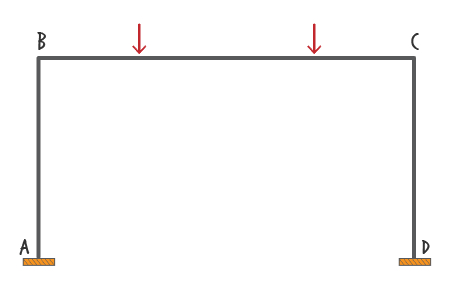Setting up the Problem ...

#### SA51: Frame Analysis Example (Matrix Displacement Method)

##### Problem Definition

Analyze the frame shown below using the Matrix Displacement Method. The frame is subjected to two concentrated member loads. There is a concentrated load of $$\small kN$$ acting on Member BC. It is located $$\small m$$ to the right of joint B. The other concentrated load has a magnitude of $$\small kN$$, and it is located $$\small m$$ to the left of joint C. Members AB and DC each has a length of $$\small m$$. The overall length of BC is $$\small m$$.

The members are made of structural steel with a modulus of elasticity ( $$\small E$$ ) of $$\small GPa$$. Members AB and CD each has a corss-sectional area ( $$\small A$$ ) of $$\small mm^2$$ and moment of inertia ( $$\small I$$ ) of $$\small mm^4$$. Member BC has a cross-sectional area of $$\small mm^2$$; and a moment of inertia of $$\small mm^4$$.Note: Move the cursor over an input field to see the valid range of values.
Note: The source of this problem can be found in this video: YOUTUBE.
Note: For a detail explanation of the Matrix Displacement Method, take our online course.